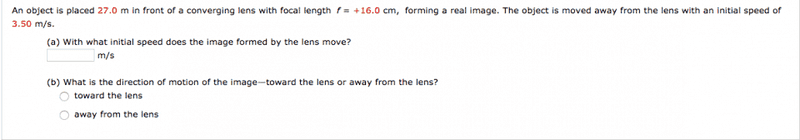# Lens question

## Homework Statement1/do+1/di=1/f

## The Attempt at a Solution

I tried finding the distance of image at 27m and then at 30.5m and taking the difference but that didn't work.

jtbell
Mentor
Does that mean you tried to find the average speed of the image? I think their talking about "initial speed" indicates that they want instantaneous speed.

ehild
Homework Helper
Your method gives the average velocity in the first second. It is a good approximation, if you calculate the image distances with enough significant digits (five at least). Show your work.

Does that mean you tried to find the average speed of the image? I think their talking about "initial speed" indicates that they want instantaneous speed.
How would I perform the calculation with instantaneous speed?

Your method gives the average velocity in the first second. It is a good approximation, if you calculate the image distances with enough significant digits (five at least). Show your work.
I tried doing this but unfortunately this didn't give me the correct answer. Is there a more accurate way of doing this?

jtbell
Mentor
How would I perform the calculation with instantaneous speed?
How do you get instantaneous speed (or more precisely, velocity) from position?

How do you get instantaneous speed (or more precisely, velocity) from position?
derivative?

jtbell
Mentor
Yup.

Yup.
Ok cool, I'm still a little confused how i would apply it to this problem however.

ehild
Homework Helper
I tried doing this but unfortunately this didn't give me the correct answer. Is there a more accurate way of doing this?
What did you get? Yes, taking the derivative of di would be more accurate, but not much different.

jtbell
Mentor
I'm still a little confused how i would apply it to this problem

You know the relationship between ##d_0## and ##d_i##: $$\frac 1 {d_o} + \frac 1 {d_i} = \frac 1 f.$$ Take the derivative with respect to t, of both sides of this equation, and you'll have a relationship between ##\frac {dd_o}{dt}## and ##\frac {dd_i}{dt}##.

jtbell
Mentor
taking the derivative of di would be more accurate, but not much different.
With Δt = 1 s I get about a 13% difference in the final answer, using the full precision of my calculator.

I agree, if Dan shows us his working, we can tell him if he at least calculated that approximation correctly.

ehild
Homework Helper
With Δt = 1 s I get about a 13% difference in the final answer.
Yes, it is true.

jtbell
Mentor
Good luck... if I want to get up for work in the morning I need to go to bed now.With Δt = 1 s I get about a 13% difference in the final answer, using the full precision of my calculator.

I agree, if Dan shows us his working, we can tell him if he at least calculated that approximation correctly.
I am getting .00011 if i do it using the approximation with average velocity. It marks this as incorrect.

ehild
Homework Helper
OK, is the image moving towards the lens, or away from it?

The method with differentiation must be more accurate. Differentiate the equation ##\frac {1} {d_o} + \frac {1} {d_i} = \frac {1} {f} ## with respect to time and solve it for di'.

jtbell
Mentor
I am getting .00011 if i do it using the approximation with average velocity.
Yes, that's what I got. Another way to get more accuracy would be to use a smaller time interval. In principle, if you make it small enough, the answer will get close enough to the exact answer to make your software happy. But you have to be very careful to avoid roundoff errors.

Yes, that's what I got. Another way to get more accuracy would be to use a smaller time interval. In principle, if you make it small enough, the answer will get close enough to the exact answer to make your software happy. But you have to be very careful to avoid roundoff errors.
Great i ended up doing this and got the right answer. Thanks!

ehild
Homework Helper
If you differentiate the equation ##\frac {1} {d_o} + \frac {1} {d_i} = \frac {1} {f}## you get ##-\frac {1} {d_o^2} d_o' -\frac {1} {d_i^2} d_i'=0##. Solve for di'.